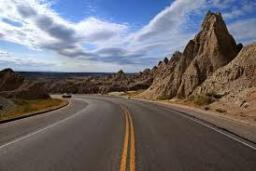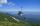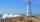# Climb

Road has climbing 1:27. How big is a angle corresponds to this climbing?

Correct result:

α =  2.12 °

#### Solution:

$\alpha =\mathrm{arctan}\left(\frac{1}{27}\right)=2.1{2}^{\circ }$We would be pleased if you find an error in the word problem, spelling mistakes, or inaccuracies and send it to us. Thank you!Tips to related online calculators

#### You need to know the following knowledge to solve this word math problem:

We encourage you to watch this tutorial video on this math problem:

## Next similar math problems:Average climb of the road is given by ratio 1:15. By what angle road average climb?
• ClimbOn the road sign, which informs the climb is 8.7%. The car drive 5 km along this road. What is the height difference that the car went to?
• Traffic signThere is a traffic sign for climbing on the road with an angle of 7%. Calculate at what angle the road rises (falls).The angle of a straight road is approximately 12 degrees. Determine the percentage of this road.
• Map - climbOn the map of High Tatras in scale 1:11000 are cable car stations in the Tatranska Lomnica and in the Skalnate Pleso with distance 354.6 mm. Altitude of this stations are 949 m and 1760 m. What is average angle of climb of this cable car track?
• BevelI have bevel in the ratio 1:6. What is the angle and how do I calculate it?
• Height differenceWhat height difference overcome if we pass road 1 km long with a pitch21 per mille?
• RhombusABCD is a rhombus, ABD is an equilateral triangle and AC is equal to 4. Find the area of the rhombus.On a straight stretch of road is marked 12 percent drop. What angle makes the direction of the road with the horizontal plane?
• RailwayRailway line had on 5.8 km segment climb 9 permille. How many meters track ascent?Between cities A and B is route 13 km long of stúpanie average 7‰. Calculate the height difference of cities A and B.Side b = 1.5, hypotenuse angle A = 70 degrees, Angle B = 20 degrees. Find its unknown sides length.What angle rising stairway if step height in 20 cm and width 26 cm?A boy of height 1.7m is standing 30m away from flag staff on the same level ground . He observes that the angle of deviation of the top of flag staff is 30 degree. Calculate the height of flag staff.From the top of a lighthouse 145 ft above sea level, the angle of depression of a boat 29°. How far is the boat from the lighthouse?Steeple seen from the road at an angle of 75°. When we zoom out to 25 meters, it is seen at an angle of 20°. What is high?From the observatory 11 m high and 24 m from the riverbank, river width appears in the visual angle φ = 13°. Calculate the width of the river.